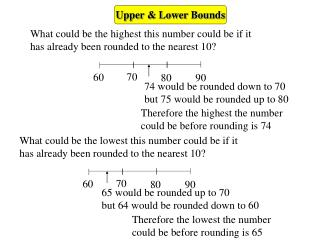DownloadDownload PresentationUpper & Lower Bounds

# Upper & Lower Bounds

Télécharger la présentation## Upper & Lower Bounds

- - - - - - - - - - - - - - - - - - - - - - - - - - - E N D - - - - - - - - - - - - - - - - - - - - - - - - - - -
##### Presentation Transcript

1. 60 60 80 80 90 90 Upper & Lower Bounds What could be the highest this number could be if it has already been rounded to the nearest 10? 70 74 would be rounded down to 70 but 75 would be rounded up to 80 Therefore the highest the number could be before rounding is 74 What could be the lowest this number could be if it has already been rounded to the nearest 10? 70 65 would be rounded up to 70 but 64 would be rounded down to 60 Therefore the lowest the number could be before rounding is 65

2. Upper & Lower Bounds This works well when counting in whole number denominations such as people or chairs or tables. When working with continuous denominations such as length, weight or height where we can have any value in a range we make an assumption. A journey of 26 miles to the nearest mile could be as long as 26.4999999…. miles or as short as 25.5 miles. It could not be 26.5 miles, as this would round to 27 miles. However, 26.4999999999…. is virtually the same as 26.5 So we overcome this difficulty by saying that 26.5 is the upper bound 25.5 is the lower bound

3. or 0.9 or 9.9 subtract from 2 1 Notice how the 0.9 disappears Upper & Lower Bounds A mathematical peculiarity: Let x = 0.999999…. 1 multiply by 10 10x = 9.999999…. 2 9x = 9 x = 1 divide by 9 It is therefore valid to give the upper bound without using recurring decimals e.g. the upper and lower bounds of 7.1 (given to the nearest 1 d.p.) are 7.15 7.05

4. Note: pence can only be whole numbers Upper & Lower Bounds Now try these • 1.Each of these quantities is rounded to the nearest whole number • of units. Write down the minimum and maximum possible size of • each quantity. • 26 g b) 4 cm c) 225 m • d) 13 litres e) 33 kg f) £249 225.5 m 224.5 m 26.5 g 25.5 g 4.5 cm 3.5 cm 12.5 g 13.5 g £249.49 £248.50 33.5 kg 32.5 kg 3.A packet weighs 2 kg, correct to the nearest 100 g. What is the maximum possible weight? 2.050 kg 5. The weight of a toffee is 5 g correct to the nearest half gram. What is the minimum possible weight of one toffee? 4.75 g

5. Upper & Lower Bounds Now try these: • Write down the upper and lower bounds of each of these • values given to the accuracy stated. • 8 m (1s.f.) 7. 300 g (1 s.f.) • 26 Kg (2.s.f) 8. 0.7 m (1 d.p.) • 25 min (2 s.f.) 9. 366 l (3 s.f.) • 2.40 m (2 d.p.) 10. 170 weeks (2 s.f.) • 0.2 kg (1 d.p.) 11. 85 g (2 s.f.) • 0.06 s (2 d.p.) 12. 210 g (2 s.f.) 8.5 7.5 350 250 26.5 25.5 0.75 0.65 25.5 24.5 366.5 365.5 2.405 2.395 175 165 85.5 84.5 0.25 0.15 215 205 0.065 0.055

6. Maximum & Minimum Values A rectangle’s sides are measured to the nearest centimetre. 26 cm 14 cm Find the largest and smallest possible areas of this rectangle Upper and lower bounds of 26 are: 26.5 cm and 25.5 cm 14.5 cm and 13.5 cm Upper and lower bounds of 14 are: = 384.25 cm2 The largest possible area = 26.5 x 14.5 = 344.25 cm2 The smallest possible area = 25.5 x 13.5

7. The table gives some information about the three counties in East Anglia Each land area is given to the nearest 100 km2 and each Population is given to the nearest 1000 people Population Land Area Population density = Calculate the lower bound or the population density for the whole of East Anglia. smallest value largest value gives the lower bound Maximum & Minimum Values 3450 669500 5450 758500 3850 661500 12750 2089500 2089500 12750 = 163.9